## calculate the electric potential 3mm from a point charge of 16Nc​

Question

calculate the electric potential 3mm from a point charge of 16Nc​

in progress 0
7 months 2021-07-22T03:10:38+00:00 1 Answers 4 views 0

1.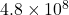volts

Explanation:

The electric potential due to a point charge is given by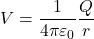where Q = charge =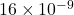C

r = distance from a point =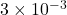m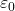= permitivity of free space

= 8.85×10^-12 C^2/N-m^2

Plugging in the numbers,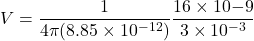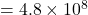volts